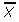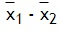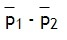# What is the Standard Error of a Sample ?

Share on

Statistics Definitions > What is the standard error?

## What is the standard error?

The standard error(SE) is very similar to standard deviation. Both are measures of spread. The higher the number, the more spread out your data is. To put it simply, the two terms are essentially equal—but there is one important difference. While the standard error uses statistics (sample data) standard deviations use parameters (population data). (What is the difference between a statistic and a parameter?).

In statistics, you’ll come across terms like “the standard error of the mean” or “the standard error of the median.” The SE tells you how far your sample statistic (like the sample mean) deviates from the actual population mean. The larger your sample size, the smaller the SE. In other words, the larger your sample size, the closer your sample mean is to the actual population mean.

### What is the SE Calculation?

How you find the standard error depends on what stat you need. For example, the calculation is different for the mean or proportion. When you are asked to find the sample error, you’re probably finding the standard error. That uses the following formula: s/√n. You might be asked to find standard errors for other stats like the mean or proportion.

Need help? Check out our tutoring page!

## What is the Standard Error Formula?

The following tables show how to find the standard deviation (first table) and SE (second table). That assumes you know the right population parameters. If you don’t know the population parameters, you can find the standard error:

• Sample mean.
• Sample proportion.
• Difference between means.
• Difference between proportions.
 Parameter (Population) Formula for Standard Deviation. Sample mean,= σ / sqrt (n) Sample proportion, p = sqrt [P (1-P) / n) Difference between means.= sqrt [σ21/n1 + σ22/n2] Difference between proportions.= sqrt [P1(1-P1)/n1 + P2(1-P2)/n2]
 Statistic (Sample) Formula for Standard Error. Sample mean,= s / sqrt (n) Sample proportion, p = sqrt [p (1-p) / n) Difference between means.= sqrt [s21/n1 + s22/n2] Difference between proportions.= sqrt [p1(1-p1)/n1 + p2(1-p2)/n2]

Key for above tables:
P = Proportion of successes. Population.
p = Proportion of successes. Sample.
n = Number of observations. Sample.
n2 = Number of observations. Sample 1.
n2 = Number of observations. Sample 2.
σ21 = Variance. Sample 1.
σ22 = Variance. Sample 2.

Check out our YouTube channel. You’ll find videos on the most popular topics. We’re adding more helpful tips every week. Comments are always welcome.

## References

Kenney, J. F. and Keeping, E. S. Mathematics of Statistics, Pt. 1, 3rd ed. Princeton, NJ: Van Nostrand, 1962.
Zwillinger, D. (Ed.). CRC Standard Mathematical Tables and Formulae. Boca Raton, FL: CRC Press, 1995.

CITE THIS AS:
Stephanie Glen. "What is the Standard Error of a Sample ?" From StatisticsHowTo.com: Elementary Statistics for the rest of us! https://www.statisticshowto.com/what-is-the-standard-error-of-a-sample/
------------------------------------------------------------------------------

Need help with a homework or test question? With Chegg Study, you can get step-by-step solutions to your questions from an expert in the field. Your first 30 minutes with a Chegg tutor is free!